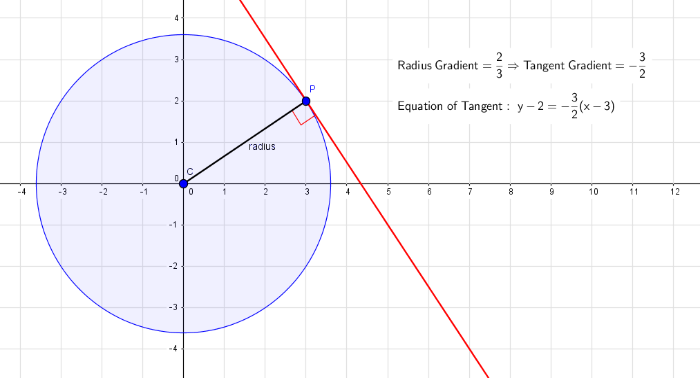# Circles

Author:
pirsquared
Topic:
Circle

## Key Facts

You must learn the following:
• The equation of a circle with centre  and radius is given by
• If the equation is given in expanded form, eg. , then it is necessary to complete the square before identifying the centre and radius:

## Relevant Circle TheoremsA tangent to the circle will meet the radius at a right-angle. ﻿(Gradient of radius is usually calculated easily from a known point on the circle, P, and the centre of the circle, C).The length from a point along a tangent can form a right-angled triangle.